# How to Calculate and Solve for Permeation | Mass Transfer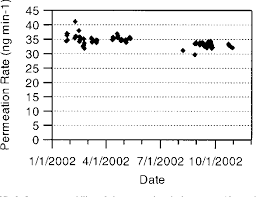The image above represents permeation.

To compute for permeation, six essential parameters are needed and these parameters are Length of Vessel (L), Diameter (D), Initial Concentration (c1), Final Concentration (c2), Inlet Radius of Pipe (r1and Exit Radius of Pipe (r2).

The formula for calculating permeation:

j = -2πLD (c1 – c2 / In(r1/r2))

Where:

j = Permeation
L = Length of Vessel
D = Diameter
c1 = Initial Concentration
c2 = Final Concentration
r1 = Inlet Radius of Pipe
r2 = Exit Radius of Pipe

Let’s solve an example;
Find the permeation when the length of vessel is 8, the diameter is 2, the initial concentration is 4, the final concentration is 9, the inlet radius of pipe is 13 and the exit radius of pipe is 11.

This implies that;

L = Length of Vessel = 8
D = Diameter = 2
c1 = Initial Concentration = 4
c2 = Final Concentration = 9
r1 = Inlet Radius of Pipe = 13
r2 = Exit Radius of Pipe = 11

j = -2πLD (c1 – c2 / In(r1/r2))
j = -2π(8)(2) (4 – 9/In(13/11))
j = (-100.53) (-5/In(1.18))
j = (-100.53) (-5/0.167)
j = (-100.53) (-29.93)
j = 3008.9

Therefore, the permeation is 3008.9 m².

Nickzom Calculator – The Calculator Encyclopedia is capable of calculating the permeation.

To get the answer and workings of the permeation using the Nickzom Calculator – The Calculator Encyclopedia. First, you need to obtain the app.

You can get this app via any of these means:

To get access to the professional version via web, you need to register and subscribe for NGN 2,000 per annum to have utter access to all functionalities.
You can also try the demo version via https://www.nickzom.org/calculator

Apple (Paid) – https://itunes.apple.com/us/app/nickzom-calculator/id1331162702?mt=8
Once, you have obtained the calculator encyclopedia app, proceed to the Calculator Map, then click on Materials and Metallurgical under Engineering.Now, Click on Mass Transport under Materials and Metallurgical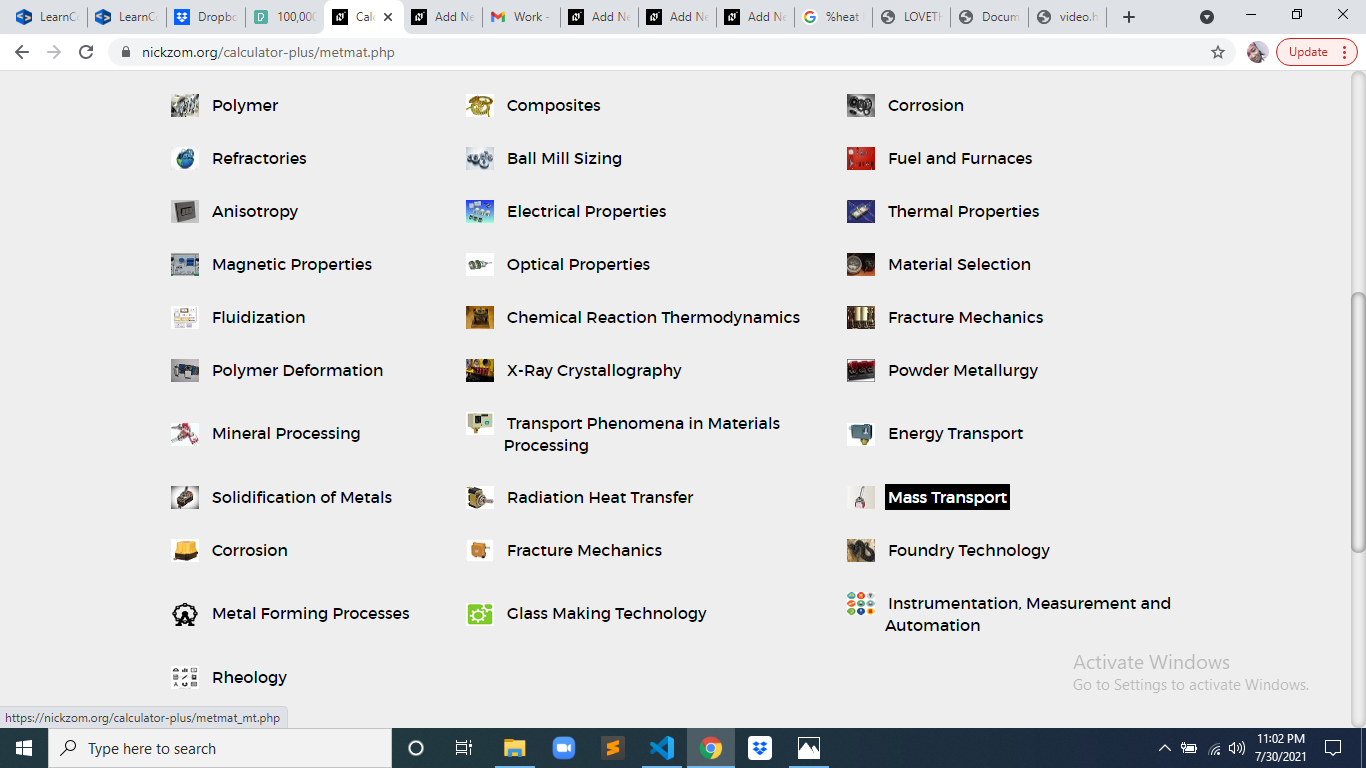Now, Click on Permeation under Mass Transport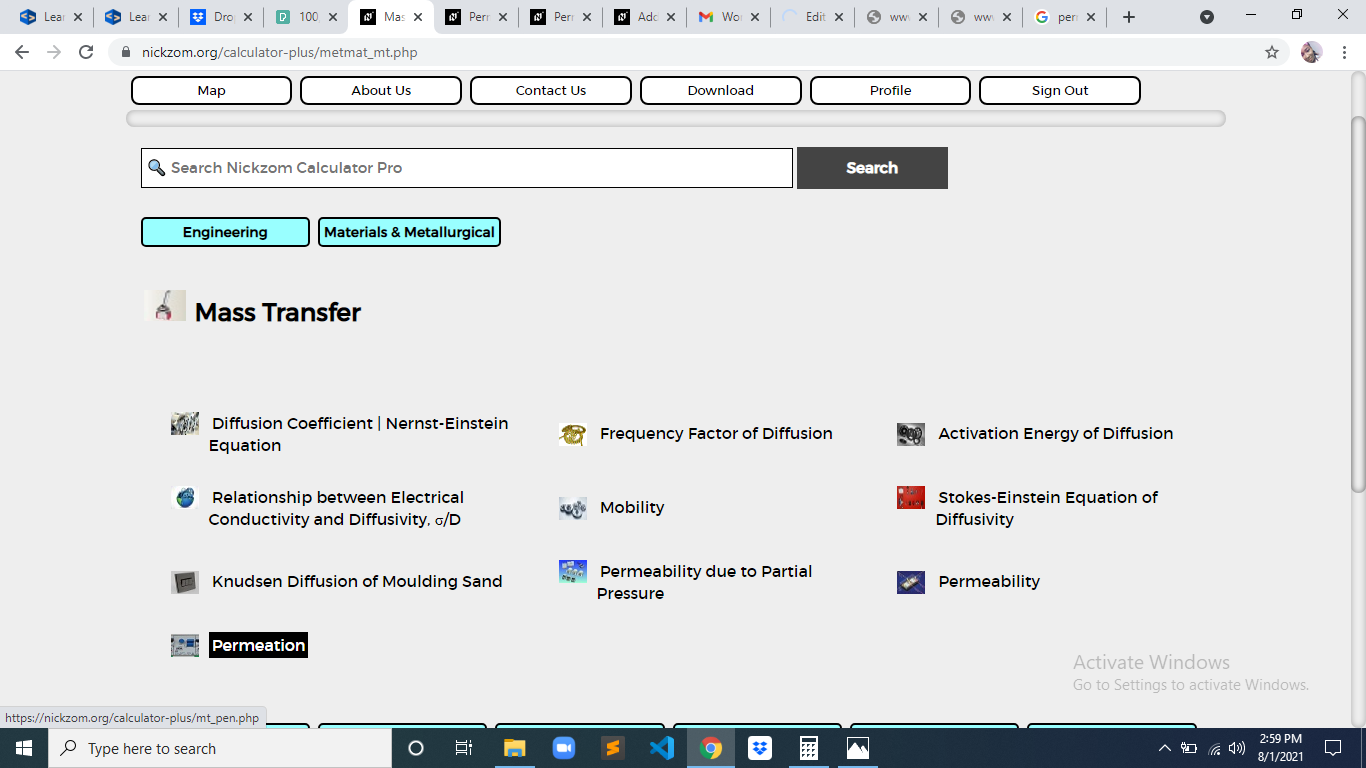The screenshot below displays the page or activity to enter your values, to get the answer for the permeation according to the respective parameter which is the Length of Vessel (L), Diameter (D), Initial Concentration (c1), Final Concentration (c2), Inlet Radius of Pipe (r1and Exit Radius of Pipe (r2).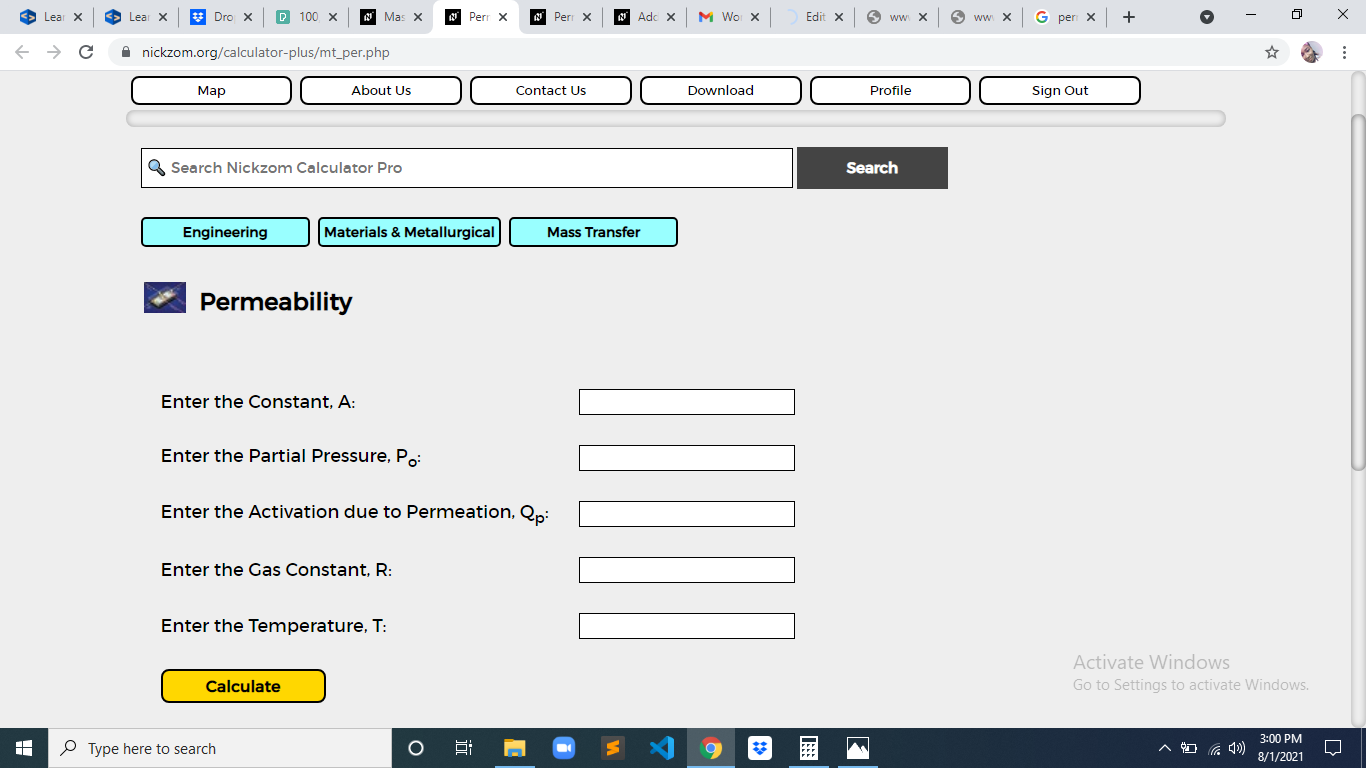Now, enter the values appropriately and accordingly for the parameters as required by the Length of Vessel (L) is 8, Diameter (D) is 2, Initial Concentration (c1) is 4, Final Concentration (c2) is 9, Inlet Radius of Pipe (r1) is 13 and Exit Radius of Pipe (r2) is 11.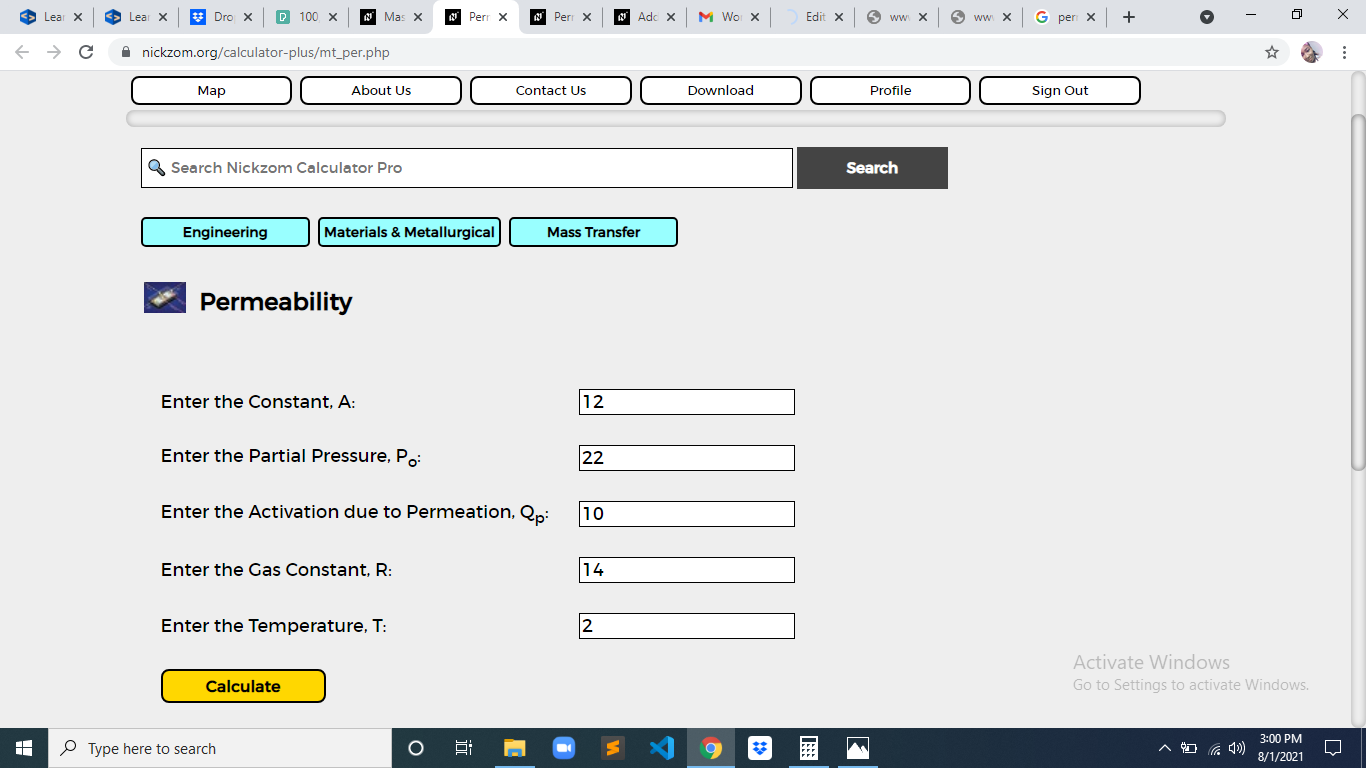Finally, Click on Calculate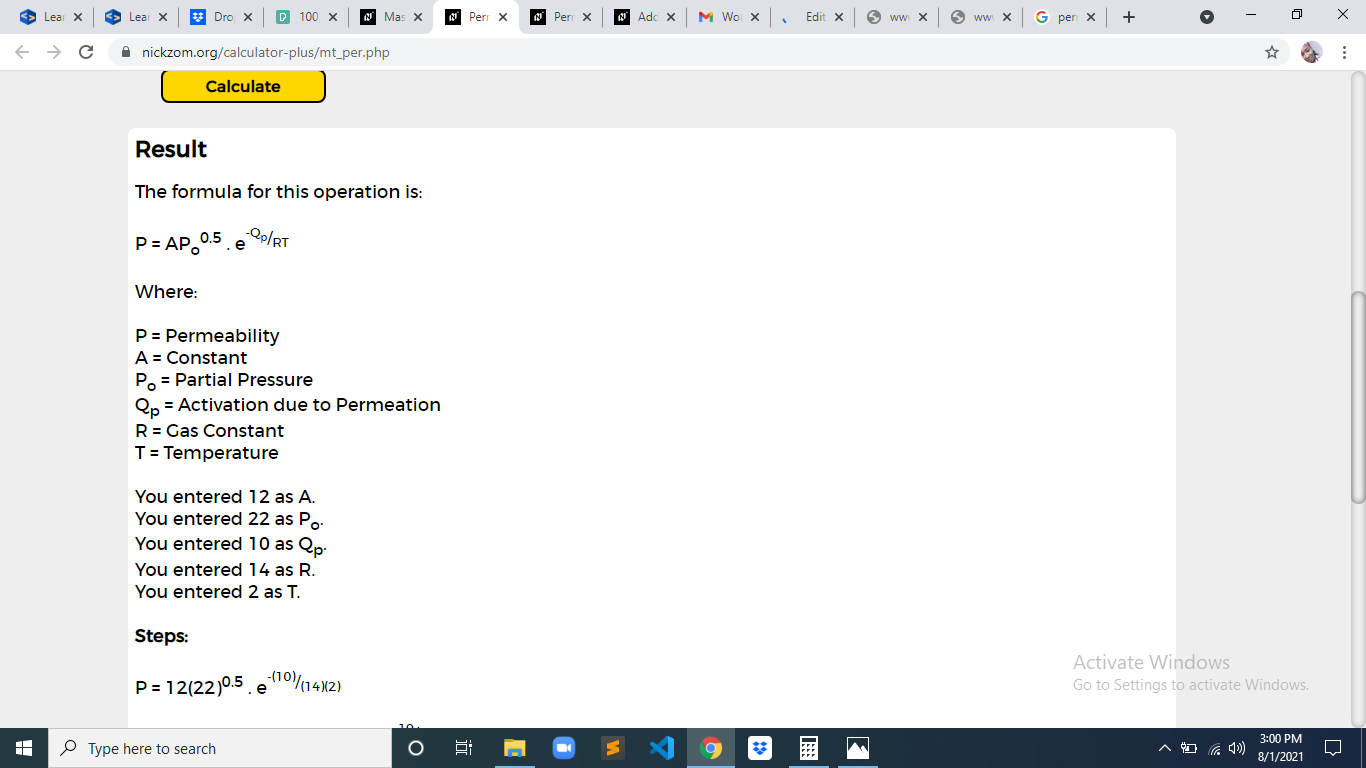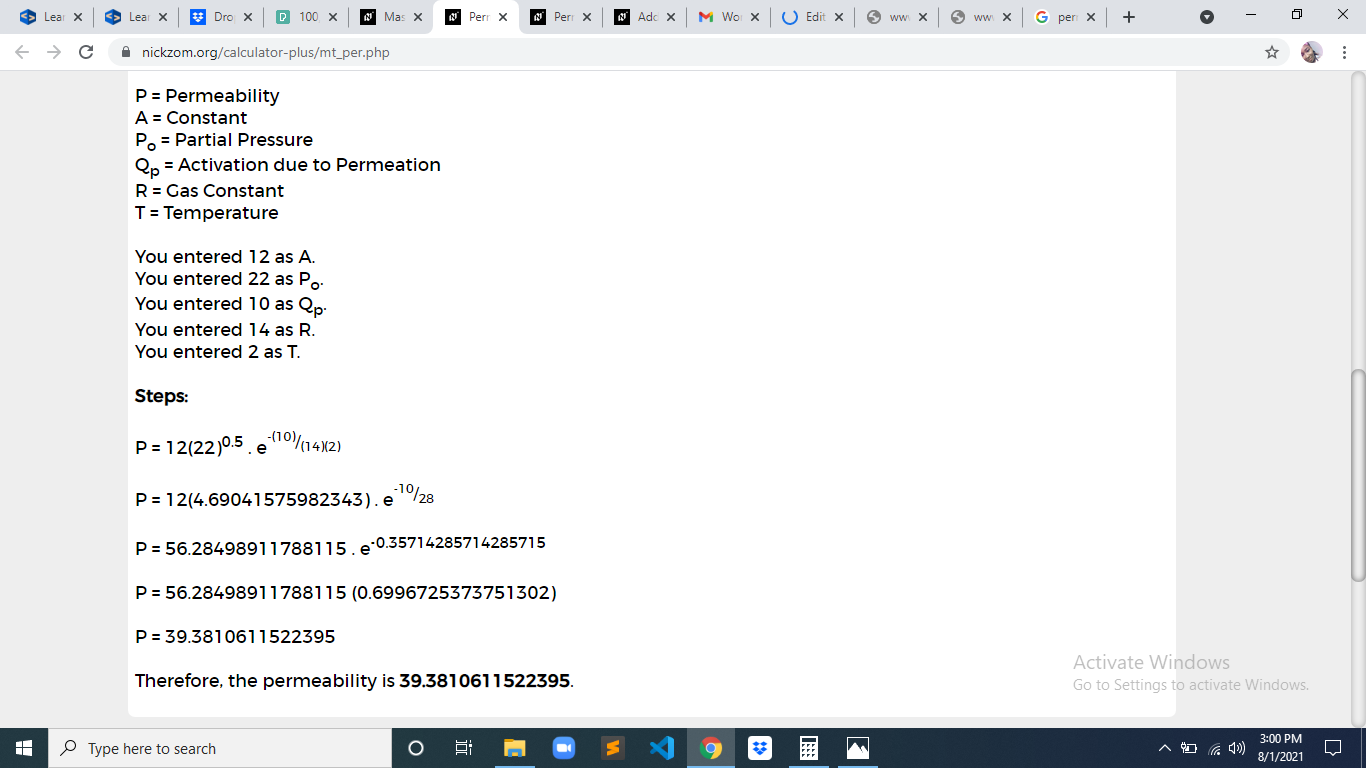As you can see from the screenshot above, Nickzom Calculator– The Calculator Encyclopedia solves for the permeation and presents the formula, workings and steps too.